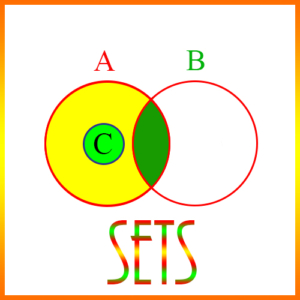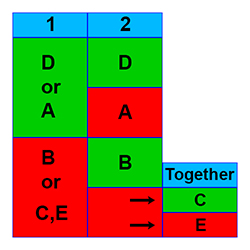# Set TheoryEvery Data Sufficiency (DS) question can have 1 of 5 possible answers, and the possible answers are the same for every DS question. All DS questions are really two questions in one. You’re given a general problem, followed by two supplemental statements. The real problem is to evaluate each of the two statements independently to determine their individual sufficiency to solve the original general problem. As you determine the sufficiency of these statements, you are able to decide which of the following 5 answers to the Data Sufficiency question is the correct answer:

A. Statement 1 is sufficient, but statement 2 is not.

B. Statement 1 is not sufficient, but statement 2 is.

C. Neither statement 1 nor statement 2 is sufficient, but together they are.

D. Both statement 1 and statement 2 are individually sufficient.

E. Neither statement 1 nor statement 2 is sufficient, and together they are not sufficient.The diagram above gives a pictorial representation of the 5 Data Sufficiency answers. The two statement numbers are shown in blue at the top of the first two columns. Note that green means sufficient, and red means not sufficient. As an example, if both statements 1 and 2 are individually sufficient, then the correct answer is D. For more information on the 5 DS answers, see our videos.

We illustrate these answers with the following examples of problems that use concepts from set theory.

Example 1)

In a random survey of V men and women voters, some were Democrats and the rest were Republicans. What is the value of V?

(1) The women surveyed outnumbered the men by a ratio of 9:8.
(2) Between 70 and 75 men were included in the survey.

Answer: C – Neither statement 1 nor statement 2 is sufficient, but together they are.

Explanation:

This problem divides the set of voters into 4 categories: Men and women Democrats, and men and women Republicans. The total number of men and women is equal to the total number of Democrats and Republicans, and this total is equal to V, the number of voters.(1) The women surveyed outnumbered the men by a ratio of 9:8.

Partial information. The ratio of women to men could help us, but without an exact number of either group we can’t compute the total number of voters. Not sufficient.

(2) Between 70 and 75 men were included in the survey.

Partial information. This does not give us an exact number of men, and it says nothing about the number of women. Not sufficient.

Alone, neither of these statements is sufficient, but together they are sufficient, because from the two statements we can see that the number of men must be divisible by 8, and 72 is the only number between 70 and 75 that is. So there are 72 men and 81 women.

Example 2)

An on-line bookstore offered a special price on each of 3 new novels. Of 1000 randomly selected customers of the bookstore, how many bought just one book?

(1) 1/2 of those surveyed bought at least one book.
(2) 50% didn’t buy a book, and 1/5 of the others bought 2 or more.

Answer: B – Statement 1 is not sufficient, but statement 2 is.

Explanation:

We can view the 1000 people in 4 categories: Those who bought 0, or exactly 1, 2, or 3 books. If we can identify the number of people in 3 of the 4 categories, we can find the number in the other one.(1) 1/2 of those surveyed bought at least one book.

Partial information. This tells us that half those surveyed were in categories 1, 2, or 3, but it gives us no further breakdown of these three categories. Not sufficient.

(2) 50% didn’t buy a book, and 1/5 of the others bought 2 or more.

Full information. The first half of the statement says half the people are in category 0. The second half says that the sum of categories 2 and 3 is 1/5 of 50%, or in other words, 10% of the people. Thus, category 1, must be 40% of the people, or 400 people. Sufficient.

Example 3)

How many products does Hansen & Slade sell in both British Columbia (BC) and Alberta?

(1) They sell 20 of their products in the two provinces, 15 of which are sold in BC and 13 in Alberta.
(2) Of the 20 products they sell there, 7 are sold only in BC, and 5 are sold only in Alberta.

Answer: D – Both statement 1 and statement 2 are individually sufficient.

Explanation:

This problem shows the typical breakdown of two overlapping sets. Some products are sold only in BC, some only in Alberta, and some in both provinces. They also have other products not sold in either province which could figure into the problem.(1) They sell 20 of their products in the two provinces, 15 of which are sold in BC and 13 in Alberta.

Full information. Since 15 and 13 total to 28, and since they only sell 20 altogether in the two provinces, then 8 of their products must be sold in both provinces. Sufficient.

(2) Of the 20 products they sell there, 7 are sold only in BC, and 5 are sold only in Alberta.

Full information. If 7 of the 20 are sold only in BC, and 5 sold only in Alberta, there must be 8 that are sold in both BC and Alberta. Sufficient.